# Alpha-beta pruning illustrated by the smothered mate

Tuesday, October 18, 2022

I've been working on Patzer, a chess engine, during my time at RC. The first engine-like thing I implemented for it was alpha-beta pruning, which is a way of pruning out branches of the search tree to significantly speed up search. This is a common algorithm, which I also implemented in my undergrad AI class. That doesn't mean that I fully understood it as I wrote it! It's pretty tricky in the details and not immediately obvious why the pruning works.

In the process of writing it and debugging it, another Recurser and I traced through the execution with a known position where we could calculate the execution. This let us figure out what was going wrong, and also gain some intuition for what the algorithm was doing. I'm going to use that same position here to illustrated alpha-beta pruning. (This is partially so that when I inevitably forget the details, I can come back here and refresh myself!)

We'll start with an overview of the algorithm viewed through the lens of the algorithm it enhances, minimax. Then we will look at the alpha-beta pruning algorithm itself. We'll wrap up by looking at our example position, a hand-constructed position which utilizes a smothered mate, and see how minimax and alpha-beta pruning work on it.

# Minimax algorithm

The base algorithm we're using here is called Minimax, and the name comes from what you're trying to do: You want to minimize the cost of the worst case maximum cost.

The intuition here is that under best play, if your opponent is making optimal moves, then they're going to make the moves which put you in the worst possible position. You're trying to pick moves which make your worst case less bad. (And that's ultimately what playing good chess is about: not making mistakes, and taking advantage of your opponent's mistakes.)

Here's Python-esque pseudocode which we could use for a basic Minimax implementation:

``````def max_step(board, depth):
if depth == 0:
return score(board)

max_score = INT_MIN;

for move in board.moves():
next_position = board.make_move(move)
score = min_step(next_position, depth - 1)
if score > max_score:
max_score = score

return max_score

def min_step(board, depth):
if depth == 0:
return -1 * score(board)

min_score = INT_MAX

for move in board.moves():
next_position = board.make_move(move)
score = max_step(next_position, depth - 1)
if score < min_score:
min_score = score

return min_score
``````

The `max_step` function is the one that corresponds to the current player: They're trying to maximize among their possible outcomes. The `min_step` function corresponds to the opponent, who is trying to minimize their opponent's best case.

(As an aside: this is usually written in the Negamax style, which reduces it down to one function. This is how I've implemented it in Patzer, but for clarity I'm presenting it as two separate functions.)

This algorithm will find all the best moves! Unfortunately, it's also slow. It exhaustively explores the entire state space of the game tree. For chess, this gets quite large quite quickly: There are over 6 trillion leaves in the minimax tree after the first 4 complete moves of the game (depth 8). My computer would not ever reach this depth.

So, what are we to do?

# Alpha-beta pruning

This is where alpha-beta pruning comes in. It's an optimization for minimax which allows us to prune out major swaths of the search tree.

The core idea of alpha-beta pruning is that there are some branches we know we won't explore, because they're too good or too bad. If a branch has a way that we can guarantee a better outcome than another branch, our opponent won't let us pursue that. If a branch has a way that our opponent can guarantee us a worse outcome than another branch, we won't go down that one, either.

To make this work, we keep track of the lower-bound (alpha) and upper-bound (beta), which let us then eliminate branches once we've confirmed that the branch will violate one of the bounds that we can otherwise guarantee. Note that this is done depth-first, like minimax. This is crucial for finding a leaf quickly to evaluate.

Here's the pseudocode of the algorithm. Again, this is the two-function implementation; you can make it one function at the expense of some readability. I've put some inline comments to highlight the differences between this and minimax. These comments are only in the max step function, but apply equally to both.

``````# we add two parameters, alpha and beta, which track lower and upper bounds
def alphabeta_max_step(alpha, beta, board, depth):
if depth == 0:
return score(board)

# note that we're not tracking the max or min anymore!
# these are tracked via alpha and beta now.

for move in board.moves():
next_position = board.make_move(move)
score = alphabeta_min_step(alpha, beta, next_position, depth - 1)

# when the score is higher than the upper bound, we just fail to the
if score >= beta:
return beta

# when we find a score that's higher than alpha, our lower bound, we
# can adopt it as the new lower bound since we know we can achieve
if score > alpha:
alpha = score

return alpha

def alphabeta_min_step(alpha, beta, board, depth):
if depth == 0:
return -1 * score(board)

for move in board.moves():
next_position = board.make_move(move)
score = alphabeta_max_step(alpha, beta, next_position, depth - 1)

if score <= alpha:
return alpha

if score < beta:
beta = score

return min_score
``````

Using these bounds turns out to be very helpful. Analysis on Chess Programming Wiki indicates that this can cut down the search tree significantly. If we always get the best move first, we would only have to evaluate 5 million positions. Obviously we can't know what the best move is or we would just play it! But there are ways we can order moves to find the best move earlier, and if you order them randomly you will still prune significantly.

# Alpha-Beta Pruning Illustrated

Alpha-beta pruning is pretty dense and hard for me to understand without tracing through a position in a game tree, so let's use an example to do that.

Here is our starting position, with white to move.

This position is set up for a classic checkmate known as the smothered mate. From here, it's forced mate in two if white finds the right moves. We chose this position as our starting position since there is a clear tactical solution which is easy to evaluate as a human, and because it's a treacherous position: If you make the wrong move, black has checkmate available as well.

We're going to look at a dramatically simplified game tree, too, to illustrate the algorithm. For this illustration, we are just considering three branches (moves are numbered starting from 1 for clarity):

1. The smothered mate line. 1. Qg8+ Rxg8 2. Nf7#
2. The "whoops I lost" line. 1. Qb7 Rc1#
3. The pawn line. 1. h3 h6 (Now no one has back rank mate) 2. h4 h5

We'll look at each of these first moves by white, followed by black's possible follow-ups, to a maximum depth of 4.

Here's our game tree, where we're examining at most 2 branches each time, except the root: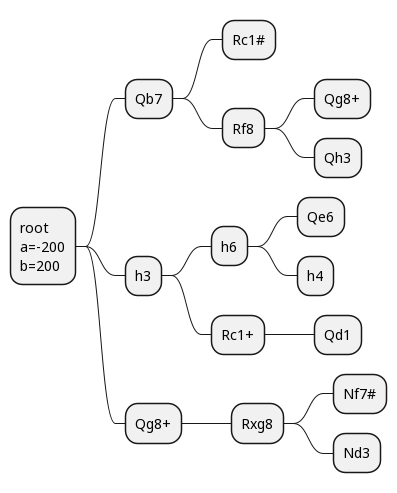This is as dramatically reduced game tree, and it's already fairly overwhelming!

(If you're not used to the notation and you're wondering what things like "Qg8+" mean, this is algebraic notation. It's not the most intuitive, but it's standard, so I think most chess players will be able to read it.)

If we look through this with minimax, we'll find one forced mate! There's another one hiding out, but it's not in our tree here, so we wouldn't find it. Since this move tree is for the player to move, the first and third layers are what we are looking at. If we find a checkmate there, great! The second layer is what our opponent is looking at, and if they find a checkmate there, boo, bad for us.

With minimax, we'd evaluate 9 leaf nodes.

Now let's consider what we'd be able to do with alpha-beta pruning. Assume we start by evaluating the Qb7 line. Then we see our opponent is able to make the move Rc1#, and that results in checkmate! This means we don't have to evaluate any further down that move tree.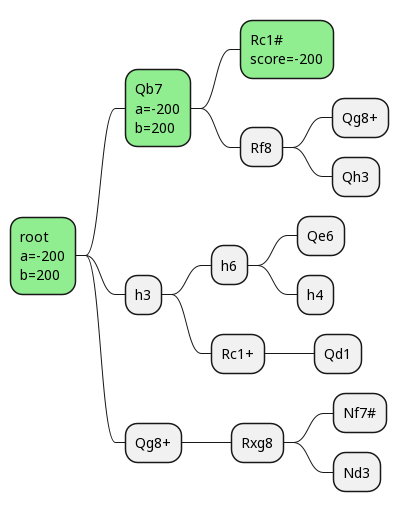We score a checkmate for ourselves as 200 points, and a checkmate for our opponent as -200.

So after we evaluate the Rc1# position as -200, that hits our `score <= alpha` case for our opponent (`alphabeta_min_step`). This returns early, and our opponent prunes out the rest of that tree, since we know that there won't be anything better than a win for our opponent. We've gotten our first pruning!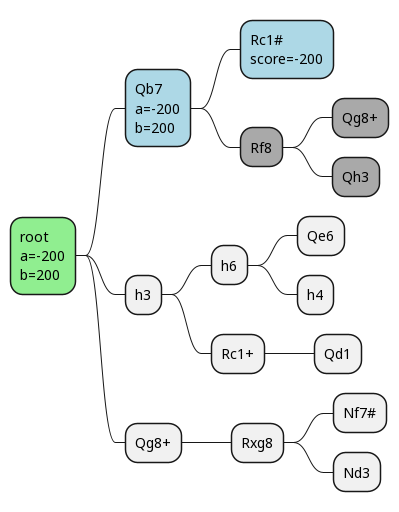This has resulted in no change to our alpha and beta values.

Now we happen to pick `h3` as our next move. We go down one line, and end up evaluating the final position as +10 (we have 17 points of material to our opponent's 7).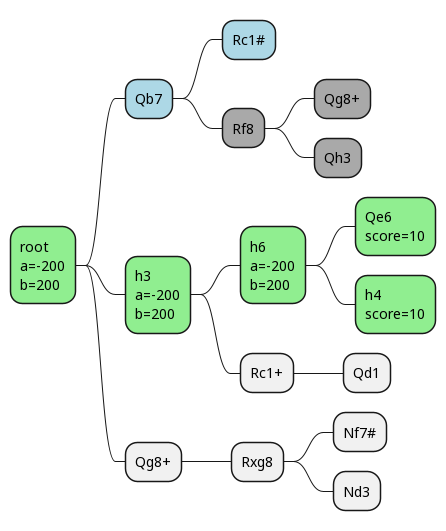Unfortunately, this doesn't result in any pruning, but it does change the alpha and beta values.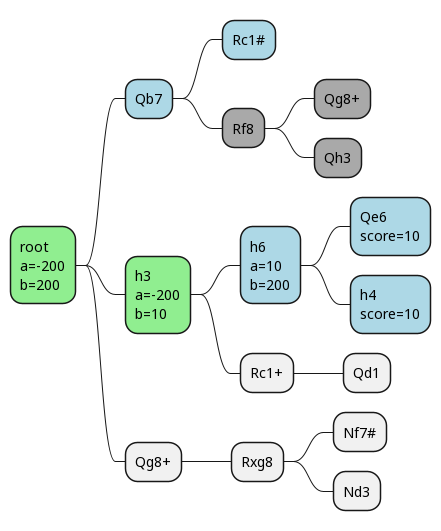We finish out this branch and have a final score for it of 10.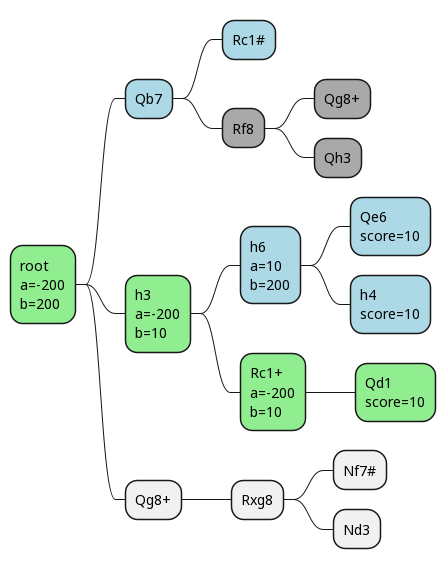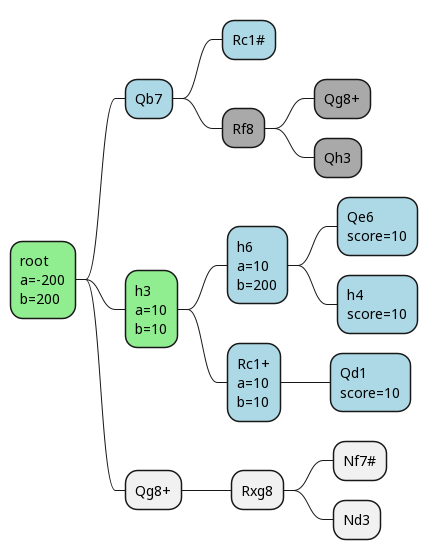Here we can see that alpha and beta have both gone to 10, so even if we had a third branch to explore after h3, we'd know the score must be 10, because the upper and lower bounds have converged! This gives white the ability to guarantee at least 10 points, so alpha changes to that at the root. It's our lower bound.

Note: there is a line in there which at depth 4 does result in checkmate for our opponent. We didn't see it. This is one of the perils of evaluating to a particular depth, and there are techniques like quiescence search which mitigate this. But we'll just pretend it isn't an issue here, and move on!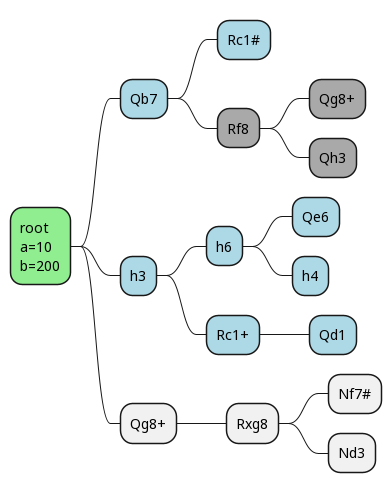Now we make our move, and our opponent's reply is forced. If we happen to then also pick the right final move first, we prune out all the remaining ones.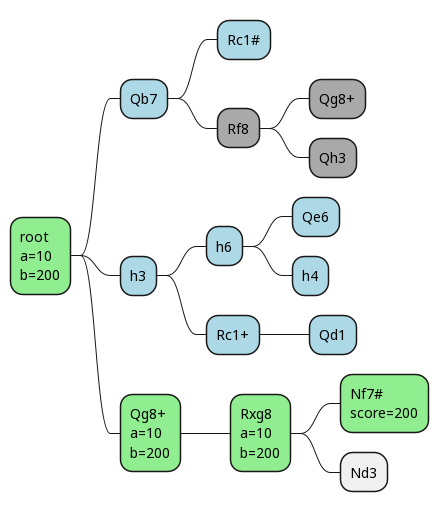Working our way back up the search tree, we can see the effects on alpha and beta.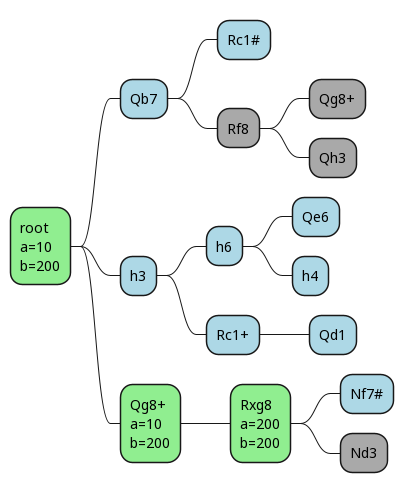Once again we've found a situation where alpha = beta, so we can prune the rest of that tree. As we work our way back up, we eventually find that this is, indeed, the best move.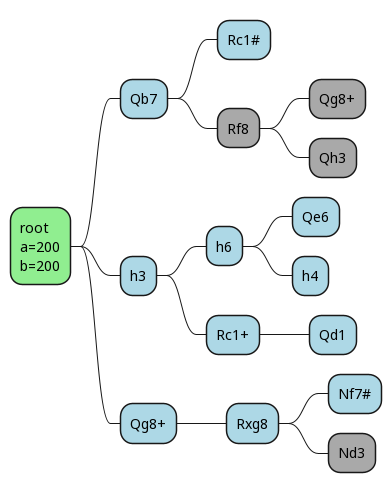The evaluation for this position is, correctly, that white is going to win.

And the best part: with alpha-beta pruning, we only had to evaluate 4 leaf nodes, instead of 7!

# I think I get it now!

This exercise was helpful for me in internalizing how alpha-beta pruning works. The fundamentals are pretty clear:

• At every round, you pick the move that maximizes your lower bound
• At every round, your opponent picks the move that minimizes your upper bound